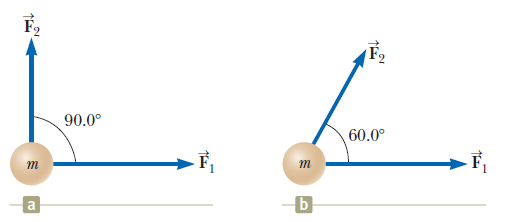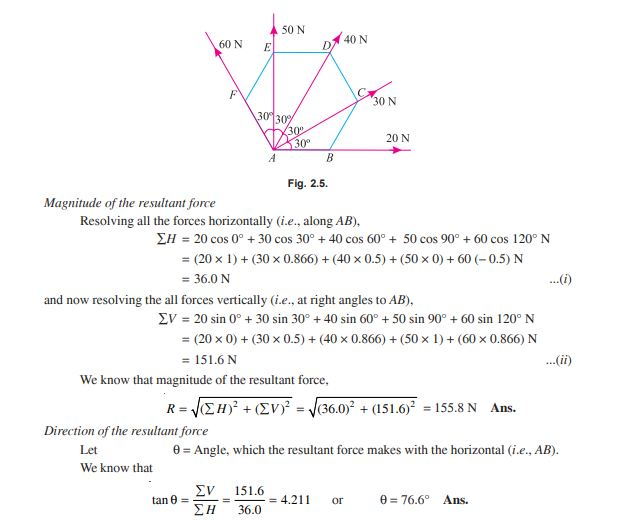• Astrophysics• Electricity• Electromagnetism• Energy• Fields• Force• Mechanics• Momentum• Nuclear Physics• Quantities & Units• States of Matter• Waves• Key ExperimentsWhen a car starts moving from rest, it gains speed because of a force acting upon it. This force is the resultant force, which combines all the other forces acting on the car. Resultant force is defined as the single force that represents the effects of all other forces on an object. A force is simply a push or pull that causes an object to change its state of motion. For example, if you want to move a box, you need to apply a force to it. The size and direction of a force can be represented by an arrow. In the figure below, you can see a force being applied to a box on a frictionless floor. The box moves in the direction of the force. Understanding resultant force is important in physics and engineering, as it helps us to better understand how objects move and interact with each other.A box being pushed across a frictionless floor by a force, F, moves in the direction of the applied force

Let's imagine that someone applies another force to the box but in the opposite direction. What would happen then? Well, if there is friction between the box and the floor, it would be harder to move the box across the floor. In the figure below, you can see this scenario, where a force (F) is pushing the box in one direction, while the friction force (f) is acting in the opposite direction. This is a common situation in our daily lives, where we need to overcome friction to move objects. By understanding the different forces acting on an object, we can predict how it will move or behave in different situations.

The resultant force is now less than before because the two forces act in opposite directions. If two forces were acting in the same direction the resultant force would be greater than before.

## Resultant force examples

The resultant force on an object is the vector sum of all of the forces acting on the object. This comes from the resultant force definition and can be illustrated with the following examples. A cart is placed on a smooth, horizontal surface and is acted upon by two forces. The first force is 30 N, acting to the right and the second force is 60 N, also acting to the right. What is the resultant force acting on the cart?

The resultant force is the combined effect - the single force that results from the two applied forces acting on the cart. In this case, the two forces act in the same direction and are therefore added. The resultant force is 90 N to the right.

A cart is placed on a smooth, horizontal surface and is acted upon by two forces. The first force is 30 N, acting to the right and the second force is 10 N, acting to the left. What is the resultant force acting on the cart?

In this scenario, the two forces are acting in opposite directions and the resultant force is now the difference between the two forces. We, therefore, subtract one force from the other to obtain a resultant force. That is, the resultant force is 20 N to the right.

A police officer is standing stationary on level ground. The weight of the officer, W, is 600 N. The reaction force that the ground exerts on the officer, R, is 600 N. Calculate the resultant force exerted on the police officer.

In this case, we can subtract one force from the other to obtain a resultant force of 0 N to the right, as follows: W - R = 600 N - 600 N = 0 N. When the resultant force on an object is 0 N, the object will remain at its current velocity unless it is acted upon by an external force.A police officer with a weight W of 800 N feels a reaction force from the ground R of 800 N. The resultant force on the police officer is zero

## Resultant force equation

When multiple forces act on an object in a straight line, we can write a general equation for the resultant force. Suppose we have two forces, F1 and F2, acting on an object in the same direction; the resultant force equation can be written as the sum of each of the forces as follows:

Resultant force = F1 + F2

On the other hand, if two forces, F1 and F2, act in a straight line but in opposite directions on an object, the resultant force equation is written as the difference between the two forces as below:

Resultant force = F1 - F2

Let's look at an example of multiple forces on an object in different directions. The corresponding resultant force diagram can be seen in the figure below.

In this example, we have three forces acting on the object: F1 to the left, F2 to the right, and F3 downwards. To calculate the resultant force, we need to add the forces in the x-direction (left and right) and the forces in the y-direction (up and down) separately.

In the x-direction, the resultant force is:

Resultant force (x-direction) = F2 - F1

In the y-direction, the resultant force is:

Resultant force (y-direction) = -F3

The negative sign indicates that the force is acting downwards. Therefore, the resultant force on the object is the vector sum of the forces in the x and y directions:

Resultant force = √[(F2 - F1)² + (-F3)²]

By calculating the magnitudes and directions of the individual forces and applying the resultant force equation, we can accurately predict the motion of the object.The resultant force on an object is the vector sum of all the forces acting on that object as shown by this diagram

andact in the same direction andacts in the opposite direction toand. The resultant force equation is, therefore:

## The magnitude of resultant force

When dealing with forces that are not parallel to the floor or surface, we need to resolve the forces into their components. This means breaking down the forces into their horizontal and vertical components, which allows us to analyze the motion of the object in each direction separately.

Let's take an example where a box is being pushed across a floor by a force of magnitude F, but the force is applied downward and to the right at an angle of 35° to the horizontal. We need to resolve the force into components, since the box moves horizontally along the surface.

To resolve the force into its components, we need to use trigonometry. We can use the following equations:

Fx = Fcosθ
Fy = Fsinθ

where Fx is the horizontal component of the force, Fy is the vertical component of the force, F is the magnitude of the force, and θ is the angle between the force and the horizontal.

In this case, we have:

Fx = Fcos35°
Fy = Fsin35°

We can then use these components to calculate the resultant force in each direction separately horizontal force is the force that causes the box to move horizontally along the surface, so we need to calculate the net force in the x-direction. The vertical force is the force that causes the box to move vertically, so we need to calculate the net force in the y-direction.

Let's assume that the box has a mass of m and there is no friction between the box and the floor. In this case, the net force in the x-direction is:

Net force (x-direction) = Fx = Fcos35°

Since there is no friction, this force is equal to the force required to accelerate the box horizontally:

F = ma
Fcos35° = ma

The net force in the y-direction is:

Net force (y-direction) = Fy - mg

where mg is the force due to gravity acting on the box.

Since the box is not moving vertically, the net force in the y-direction is zero:

Fsin35° - mg = 0
Fsin35° = mg

We can use these equations to solve for F and find the magnitude of the force required to move the box. Once we have the magnitude of the force, we can use the components to find the direction of the force and the resultant force on the box.A force F is applied to a box at an angle of 35° to the horizontal surface. The box moves horizontally along the surface

We can draw a resultant force diagram to resolveinto a horizontal and vertical component as follows (not drawn to scale).A 20 N force is resolved into its horizontal (16 N) and vertical (11 N) components

Great summary! Here are some additional key takeaways:

• Forces are vector quantities that have both magnitude and direction.
• To analyze the motion of an object, we need to consider the net force acting on the object.
• The net force is the vector sum of all the forces acting on the object.
• When forces are not parallel to the surface, we need to resolve them into their horizontal and vertical components using trigonometry.
• The horizontal component of the force is responsible for causing the object to move horizontally along the surface.
• The vertical component of the force is responsible for balancing the normal contact force between the object and the surface.
• If the net force on an object is zero, the object will remain at rest or continue moving with constant velocity (if it was already in motion).
• If the net force on an object is nonzero, the object will accelerate in the direction of the net force.
• The magnitude of the acceleration is proportional to the magnitude of the net force and inversely proportional to the mass of the object (F = ma).

By understanding these concepts, we can accurately predict the motion of objects in a variety of situations and apply them to real-world scenarios.

## Resultant Force

What is resultant force?

We call the single force that represents the effects of all of the other forces acting on an object, the resultant force.

How to calculate the resultant force?

The resultant force on an object is the vector sum of all the forces acting on that object.

How to find the resultant force of 3 vectors?

The resultant force is the vector sum of the three forces acting on that object.

How to find angle of resultant force?

The tan of the angle between the resultant force and its horizontal component is equal to the vertical component of the resultant force divided by its horizontal component.

What is the formula for resultant force?

FR = F1 + F2 + F3 + ... + Fn14-day free trial. Cancel anytime.Join 10,000+ learners worldwide.The first 14 days are on us96% of learners report x2 faster learningFree hands-on onboarding & supportCancel Anytime